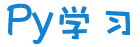注册    登录创作新主题

Python
 python开源   Django   Python   DjangoApp   pycharm
DATA
 docker   Elasticsearch

 问与答   闲聊   招聘   翻译   创业   分享发现   分享创造   求职   区块链   支付之战
aigc
 aigc   chatgpt
WEB开发
 linux   MongoDB   Redis   DATABASE   NGINX   其他Web框架   web工具   zookeeper   tornado   NoSql   Bootstrap   js   peewee   Git   bottle   IE   MQ   Jquery

Python88.com
 反馈   公告   社区推广

Py学习  »  Python

# 如何在 Python 中使用断点调试

Crossin的编程教室 • 3 月前 • 146 次点击

``def twice(n):``    n *= 2``    return n``a = int(input("a:"))``b = int(input("b:"))``if a > 3:``    b += 4``    if b > 5:``        c = a + twice(b)``    else:``        c = twice(a) + b``else:``    b -= 2``    if b < 1:``        c = a - twice(b)``    else:``        c = twice(a) - b``print(c)``

````def twice(n):``    n *= 2``    return n``a = int(input("a:"))``b = int(input("b:"))``print("====a,b:", a, b)``if a > 3:``    b += 4``    print("====1 b:", b)``    if b > 5:``        c = a + twice(b)``        print("====1 c:", c)``    else:``        c = twice(a) + b``        print("====2 c:", c)``else:``    b -= 2``    print("====2 b:", b)``    if b < 1:``        c = a - twice(b)``        print("====3 c:", c)``    else:``        c = twice(a) - b````        print("====4 c:"

, c)````print(c)````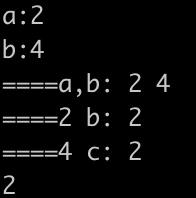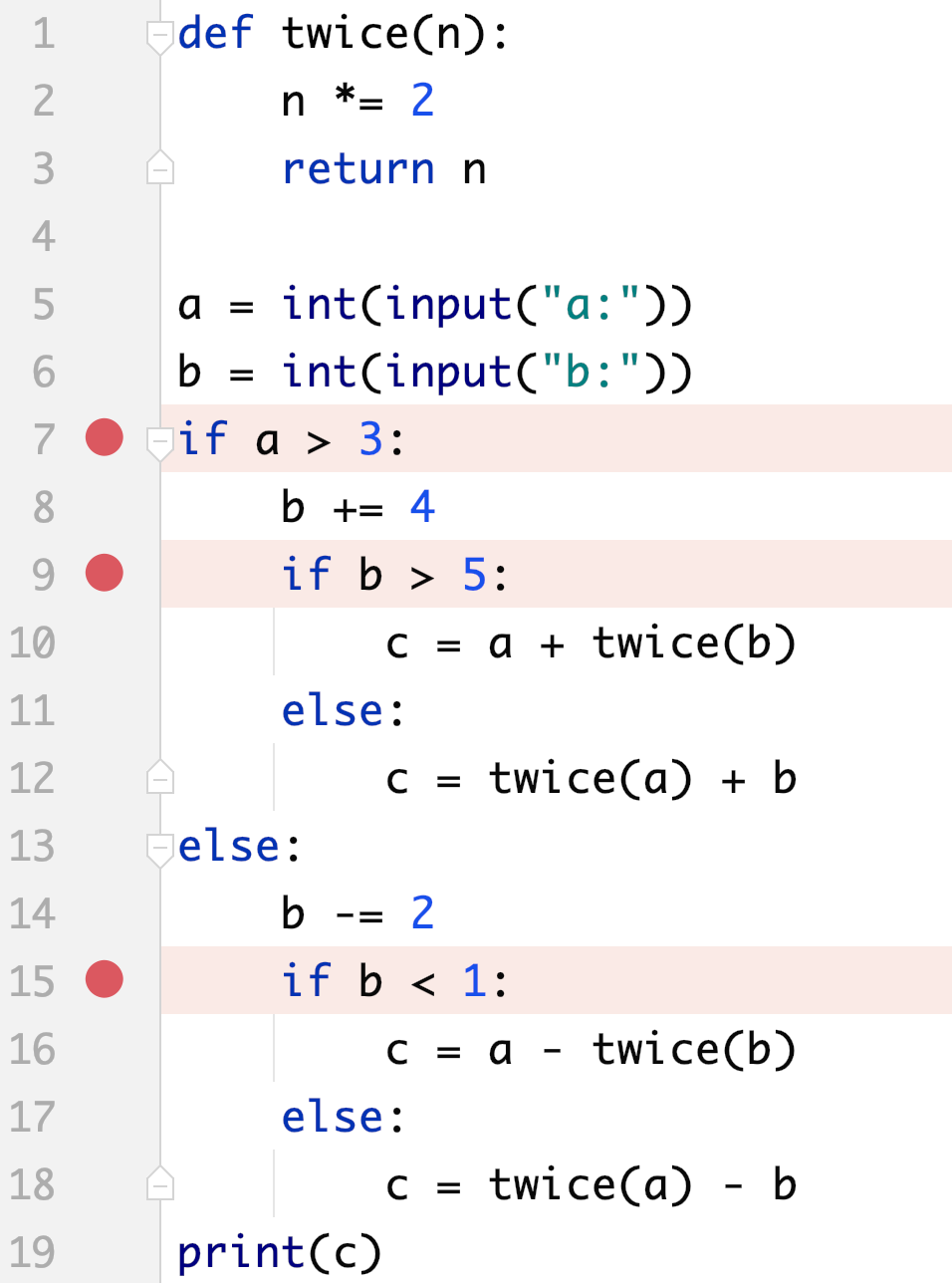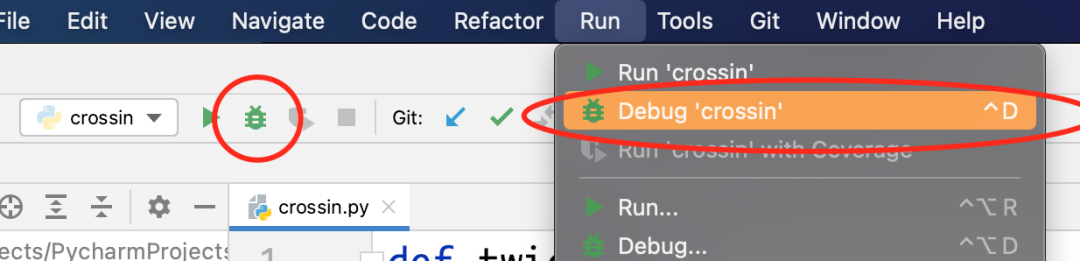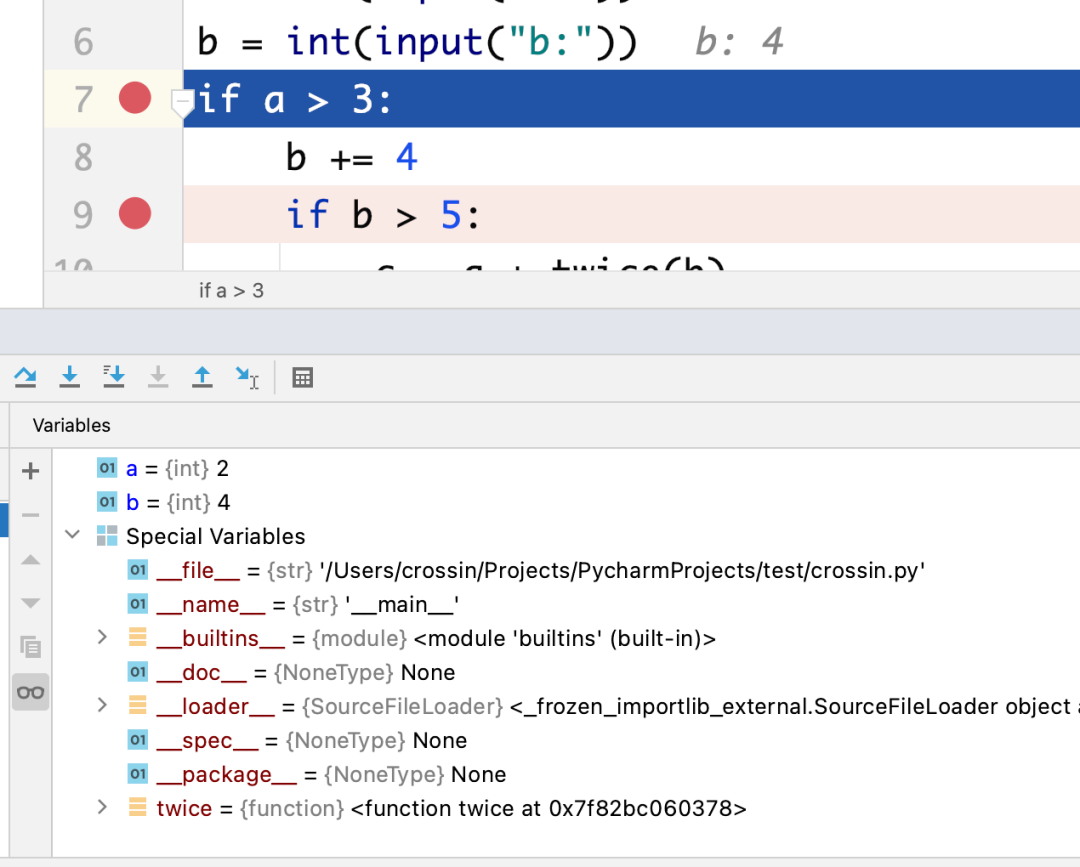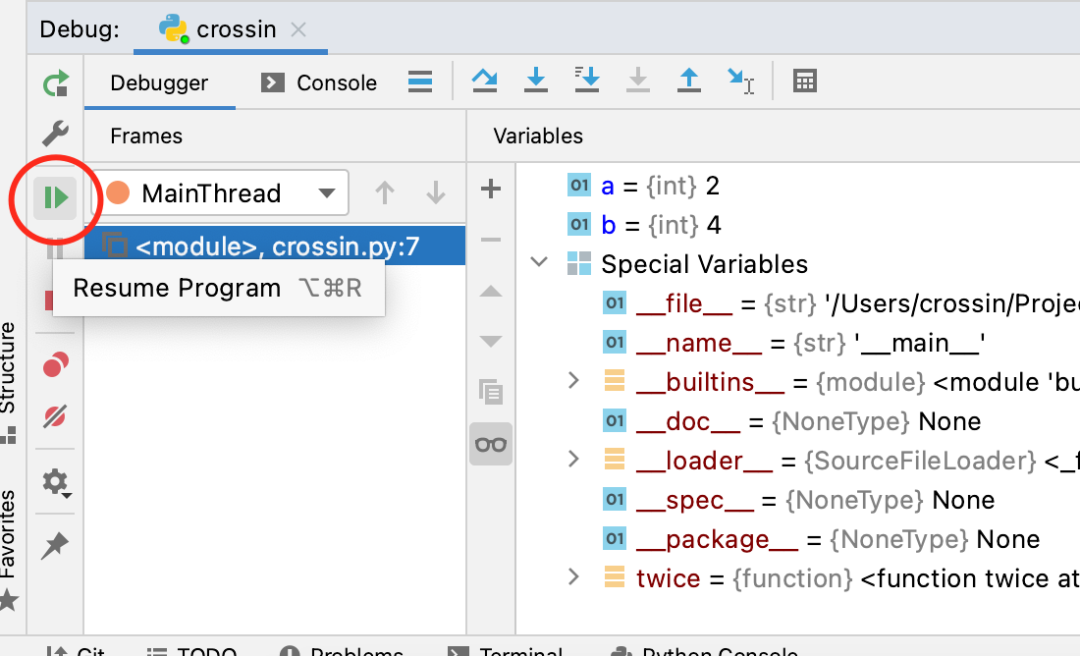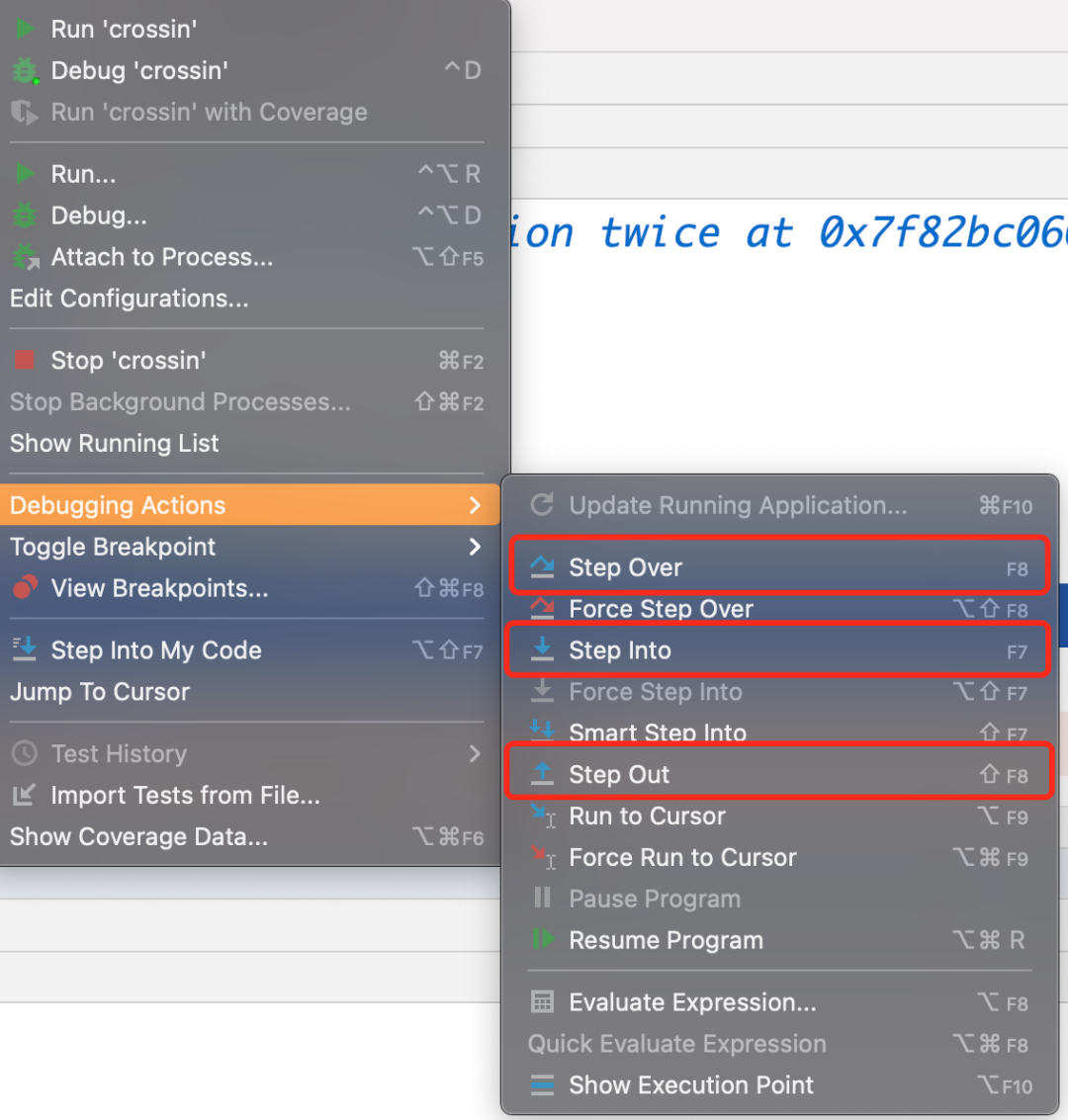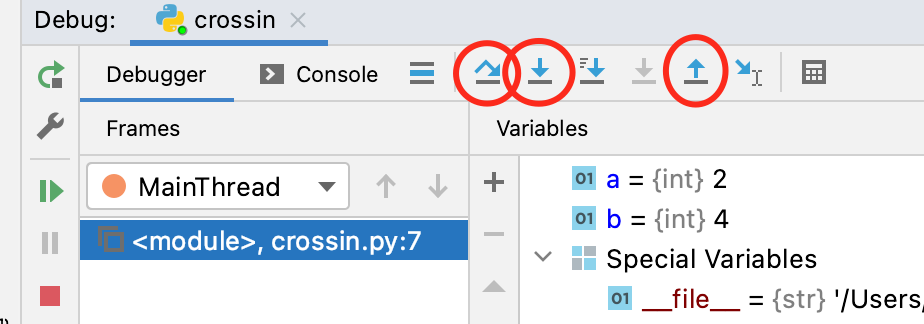“工欲善其事，必先利其器”。把上述调试工具熟悉之后，对你的开发效率将会有很大提升。

Crossin的新书码上行动：零基础学会PYTHON编程（CHATGPT版）已经上市了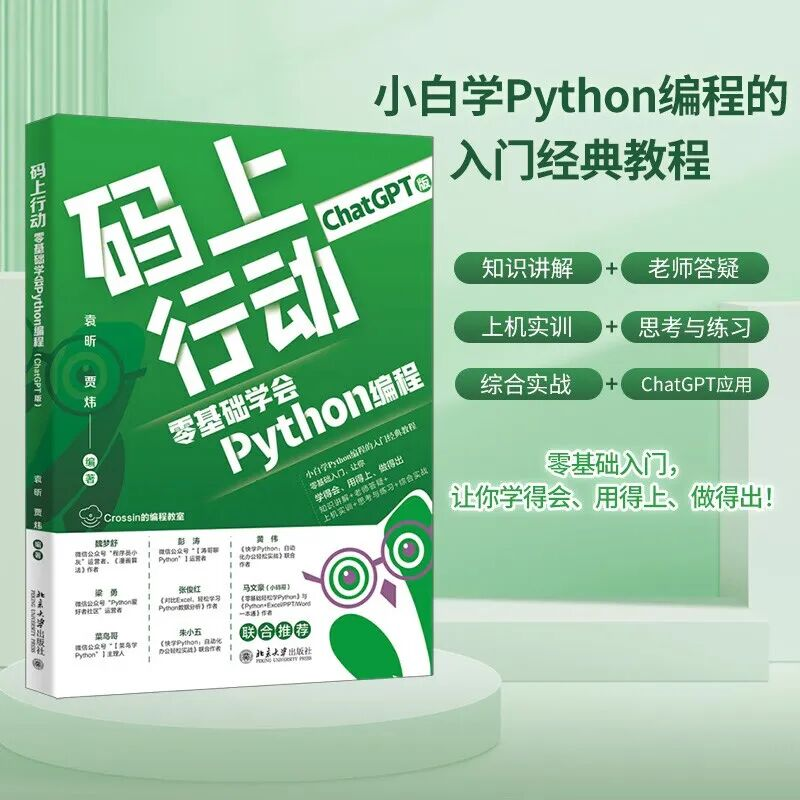_往期文章推荐_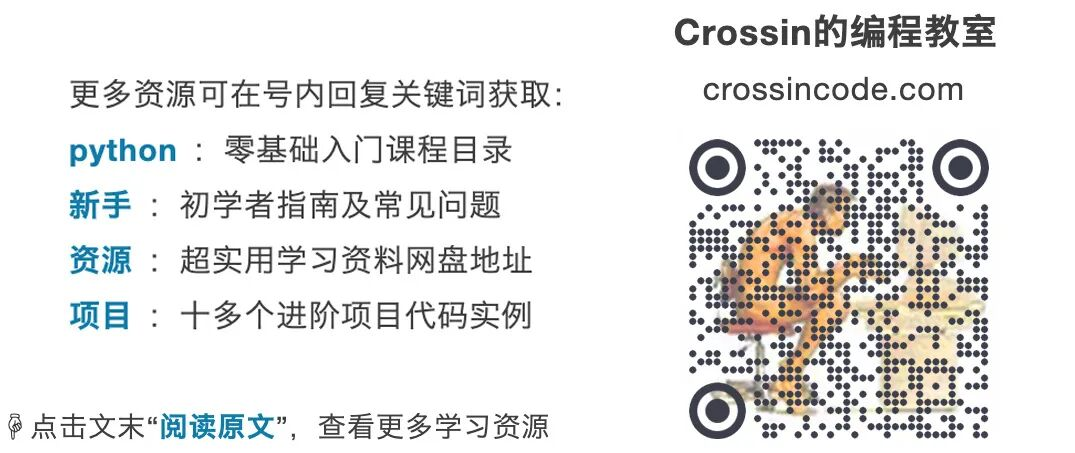Python社区是高质量的Python/Django开发社区